# [Scala MOOC II] Lec 3: Functions and State

This week: scala for imperative programming.

# Lecture 3.1 - Functions and State

So far: pure functional programming
→ side-effect free: therefore time doesn't matter.
Any rewriting that terminates lead to the same solution. (Churcher-Rosser Th)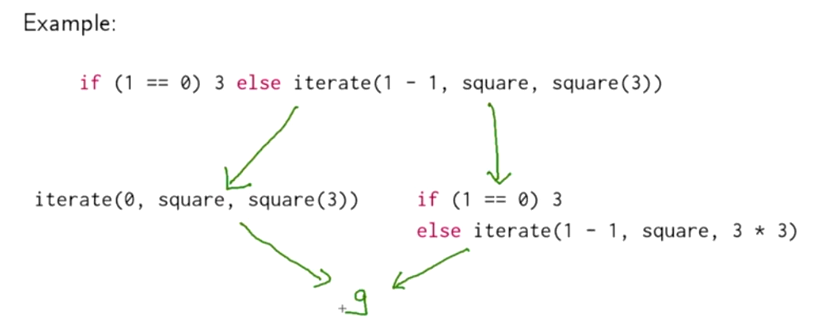Now: mutable states

Stateful objects: objects can have state that change over time. (state is influenced by its history). ⇒ variables `var` in scala, associates a value to a name, and can be changed by assignment.

ex. bank account — pretty much like java class

```class BankAccount{
private var balance = 0
def deposit(amount:Int): Unit =
if (amount>0) balance = balance + amount
def withdraw(amount:Int): Unit =
if(0<amount && amount<=balance){
balance = balance - amount
balance
}else throw new Error("insufficient balance")
}

val acct = new BankAccount
acct deposit 50
acct withdraw 20
acct withdraw 10
```

ex2. streams impolemented using mutable variable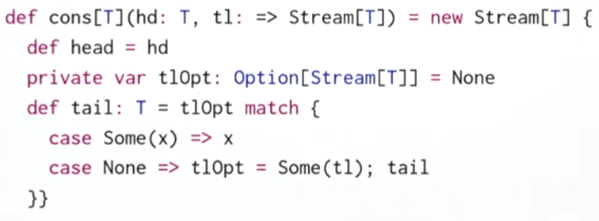# Lecture 3.2 - Identity and Change

when are 2 (mutable) objs equal ? → what is equal?

`x` and `y` are operationally equivalent if no possible test can distinguish between them.

to test if `x` and `y` are the same: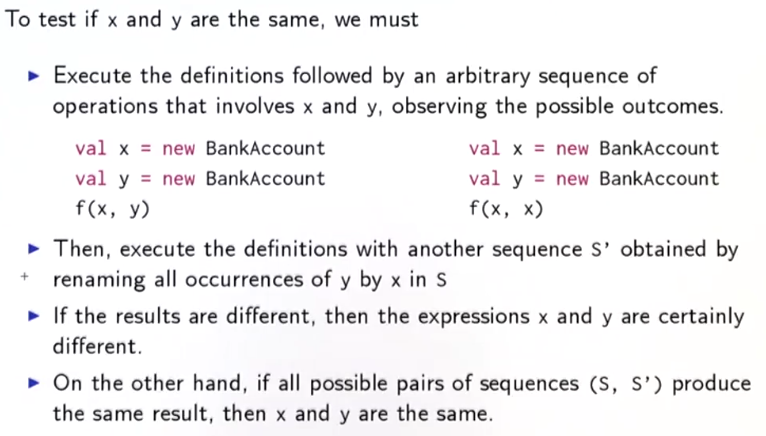The substitution model is no longer valid:

• x and y are `not` the same:

val x = new BankAccount
val y = new BankAccount

• x and y are the same:

val x = new BankAccount
val y = x

# Lecture 3.3 - Loops

prop: `var`s are already enough to model all imperative programs. Can model loops using functions.

ex. scala while loop

```def power (x:Double, exp:Int): Double = {
var r = 1.0; var i = exp
while(i>0) {r = r*1; i = i-1}
r
}
```

This while loop can be implemented using a function `WHILE`:

```def WHILE(cond: => Boolean)(cmd: =>Unit):Unit = {// cond and cmd must be passed by name
if(cond) {
comd
WHILE(cond)(cmd)
}
else () // or `()`=Unit (= void in java)
}
```

exercice: write a REPEAT function: `REPEAT{cmd} (condition)` , similar to `do...while`

```def REPEAT(cmd: =>Unit)(cond: =>Boolean):Unit = {
cmd
if (cond) () // stop
else REPEAT(cmd)(cond)
}
```

do-while loop syntax in scala: `do{cmd}while(cond)`

the classical `for` loop in java can NOT be modeled by higher-order function, because the for loop arguments contains declaration of a variable i. However, in scala, use:

`for(i <- 1 until 3) println(i)`

This is similar to previously discussed `for-expression`, but using `foreach` instead of `map`/`flatMap`.

example:

`for(i<-i until 3; j<- "abc") println(i+" "+j)`

translates to:

`(1 until 3) foreach (i => "abc" foreach (j => println(i+" "+j)))`

# Lecture 3.4 - Extended Example: Discrete Event Simulation

digital circuit simulator.
A digital circuit(DC) is composed of `wires` and functional components.

Basic components: Inverter, AND gate, OR gate
components have reaction time (delay)

diagrams:example: half adder (input=a,b, output=sum and carry)language to describe digital circuits: using classes and functions

```val a,b,c = new Wire
def inverter(input: Wire, output:Wire): Unit
def andGate(a1: Wire, a2: Wire, output:Wire): Unit
def orGate(a1: Wire, a2: Wire, ouput:Wire): Unit
```

a half adder can be defined as:

```def halfAdder(a: Wire, b: Wire, s: Wire, c:Wire): Unit = {
val d,e = new Wire
orGate(a,b,d)
andGate(a,b,c)
inverter(c,e)
andGate(d,e,s)
}
```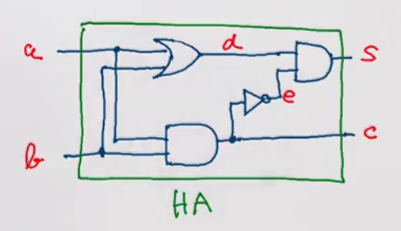And this half adder can be used as another component, for example, for full adder: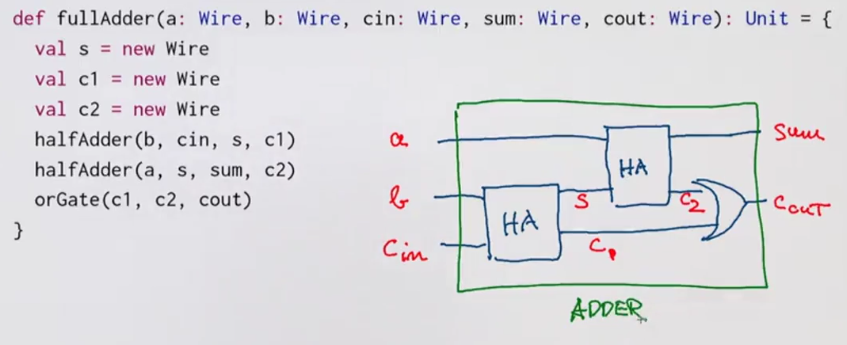# Lecture 3.5 - Discrete Event Simulation: API and Usage

give implementations of the digital circuits, based on an API for discrete event simulation.

### discrete evenet simulator

performs actions, specified by user at a given moment.

An `Action`: a function that takes 0 parameters and returns `Unit`.

`type Action = () => Unit`

class hierachy: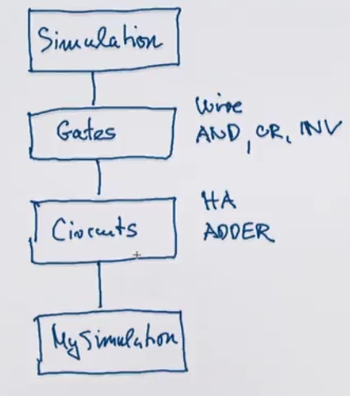```trait Simulation {
def currentTime: Int = ???
def afterDelay(dalay: Int)(block: =>Unit): Unit = ???
def run(): Unit = ???
}
abstract class Gates extends Simulation{
class Wire{...}
...}
abstract class Circuits extends Gates{...}
object sim extends Circuits
...
```

`Wire` class:
state of a wire is modeled by 2 private vars

• `getSignal: Boolean`: current value of signal in wire
• `setSignal(sig:Boolean):Unit` : modifies value of signal
• `addAction(a: Action): Unit`: attach actions to be executed at each change of signal
```class Wire{
private var sigVal = false
private var actions: List[Action] = List()
def getSignal = sigVal
def setSignal(sig:Boolean):Unit =
if(getSignal!=sig){
sigVal = sig
actions foreach (_()) // for(a<-actions) a()
}
actions = a::actions
a() // have to perform it when added
}
}
```

`Inverter`:install an action on its input wire, the change is effective after a delay.

```def inverter(input:Wire, output:Wire):Unit = {
def invertAction():Unit = {
val inputSig = input.getSignal
afterDelay(InverterDelay) {output setSignal !inputSig}
}
}
```

`andGate/orGate`is similar: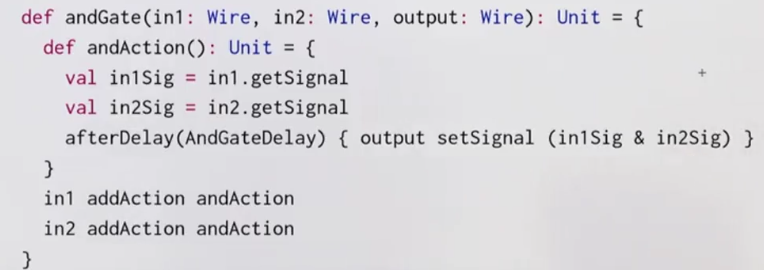# Lecture 3.6 - Discrete Event Simulation: Implementation and Test

implement the simulation trait: keep each instance of `Simulation` in `agenda` of actions to perform.

`Agenda` is a list of `Event`s, each event consists of an `action` and the `time`, sorted by actions' time.

To run the simulation, use a `loop` to handle events in agenda.

To examine the changes of the signals in wires, use funciton `probe`.

```trait Simulation {
type Action = ()=>Unit
case class Event(time:Int, action:Action)
private type Agenda = List[Event]
private var agenda: Agenda = List()
private var curtime = 0
def currentTime: Int = curtime
def afterDelay(delay: Int)(block: =>Unit): Unit = {
val item = Event(currentTime+delay, ()=>block)
agenda = insert(agenda, item) //insert to the write time
}
private def insert(ag:List[Event], item:Event): List[Event] = ag.match{
case first::rest if first.time<=item.time
=> first::insert(rest, item)
case _
=> item::ag
}
private def loop():Unit = // event handling loop
agenda match{
case first::rest =>
agenda = rest
curtime = first.time
first.action()
loop()
case Nil =>
}
def run(): Unit = {
afterDelay(0){println(s"*** simulation started, time = \$currentTime ***")}
loop()
}
def probe(name:String, wire:Wire):Unit = {
def probeAction(): Unit =
println(s"\$name time = \$currentTime, value = \${wire.getSignal}") // string formatting in scala
}
}
```

to pack delay constrains into their own trait, use `extend..with..` syntax:

```trait Parameters{
def InverterDelay = 2
def AndGateDelay = 3
def OrGateDelay = 5
}
object sim extends Circuits with Paramters
```

### summary

state and assignments make model more complicates, lose referential transparency
on the other hand, assignments allow formulate certain programs in an elegant way.

# Programming Assignment: Quickcheck

This assignment has nothing to do with the mutable data... but rather to use scalacheck for testing.

Write properties that a heap should have to test heap implementations.